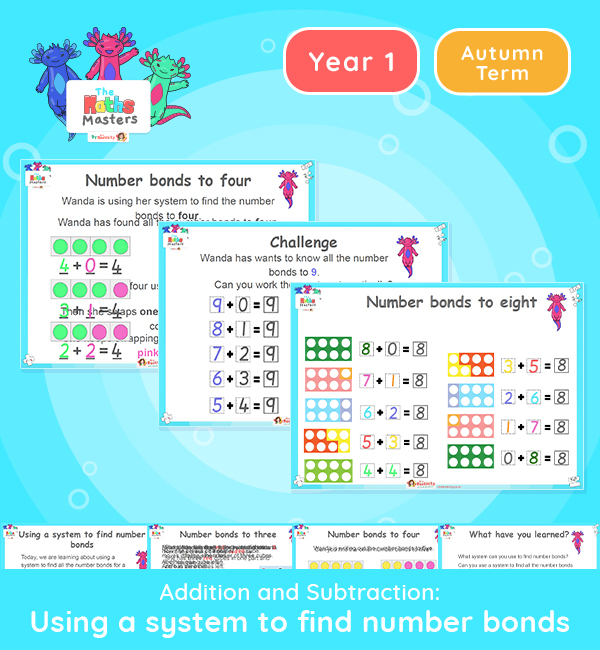# Year 1 | Using a System to Find Number Bonds Lesson Presentation## White Rose year 1 addition and subtraction resources

This lesson introduces number bonds to 10 and how this can help with addition.

Aligned with the White Rose Maths scheme of work, this Year 1 | Using a System to Find Number Bonds Lesson Presentation is fully editable and designed for the Year 1 maths curriculum to cover the following curriculum objectives:.

This is one of a series of lessons on number bonds. It focuses on systematically finding all the number bonds for a number up to 10.

White Rose Maths Small Steps: Systematic methods for number bonds within 10.

NC Links: Read, write and interpret mathematical statements involving addition (+), and equals (=) signs. • Add one digit numbers to 10, including zero. • Solve one step problems that involve addition using concrete objects and pictorial representations *• Represent and use number bonds within 10

Ready-to-progress criteria:  1AS-1 Compose numbers to 10 from 2 parts, and partition numbers to 10 into parts.

Previous Experience: Understand the cardinal value of number words. Subitise for up to 5 items. Automatically show a given number using fingers.

1NF-1 Develop fluency in addition facts within 10.

Previous experience: Being to experience partitioning and combining numbers within 10.

TAF Statements: Working Towards Add and subtract (one digit numbers) explaining their method verbally in pictures or using apparatus.

Aligned with the order of teaching of the White Rose Maths scheme of work, use this to help your pupils get to grips with each mathematical concept. This lesson presentation also includes varied fluency activities, problem solving, and mathematical discussion questions too.

Explore our other year 1 addition and subtraction resources.